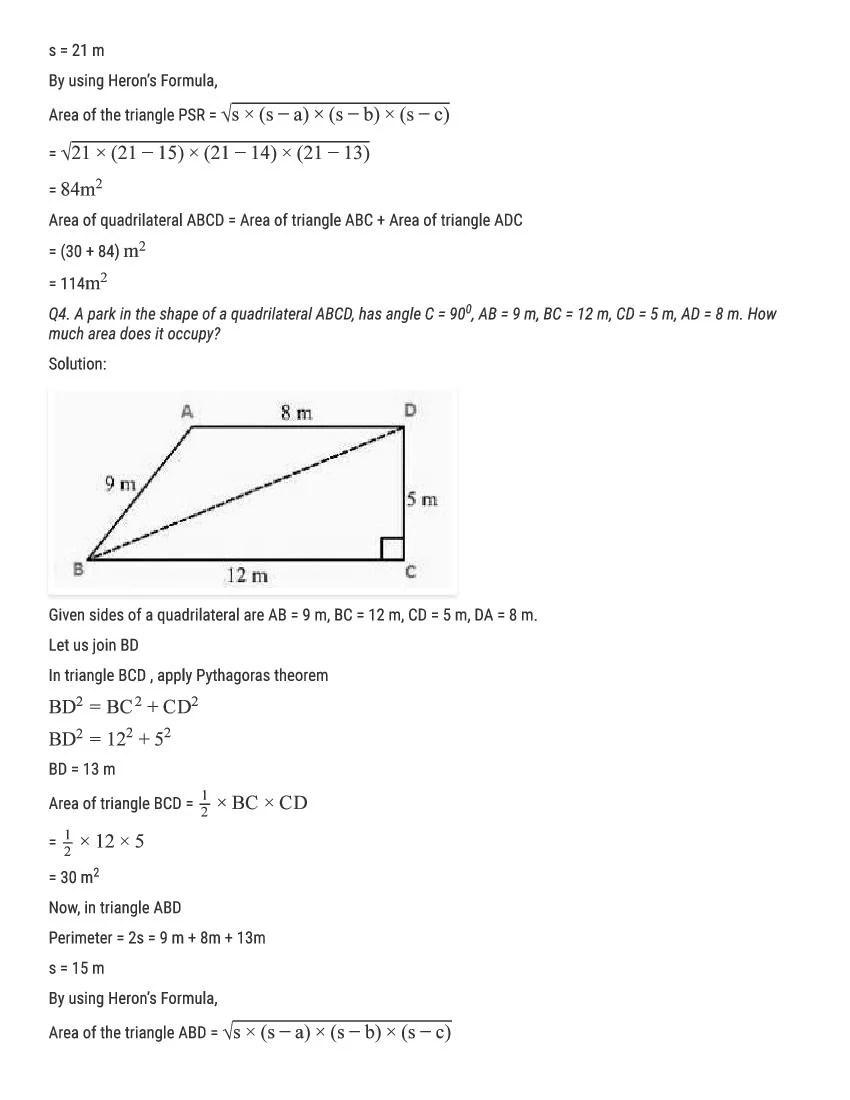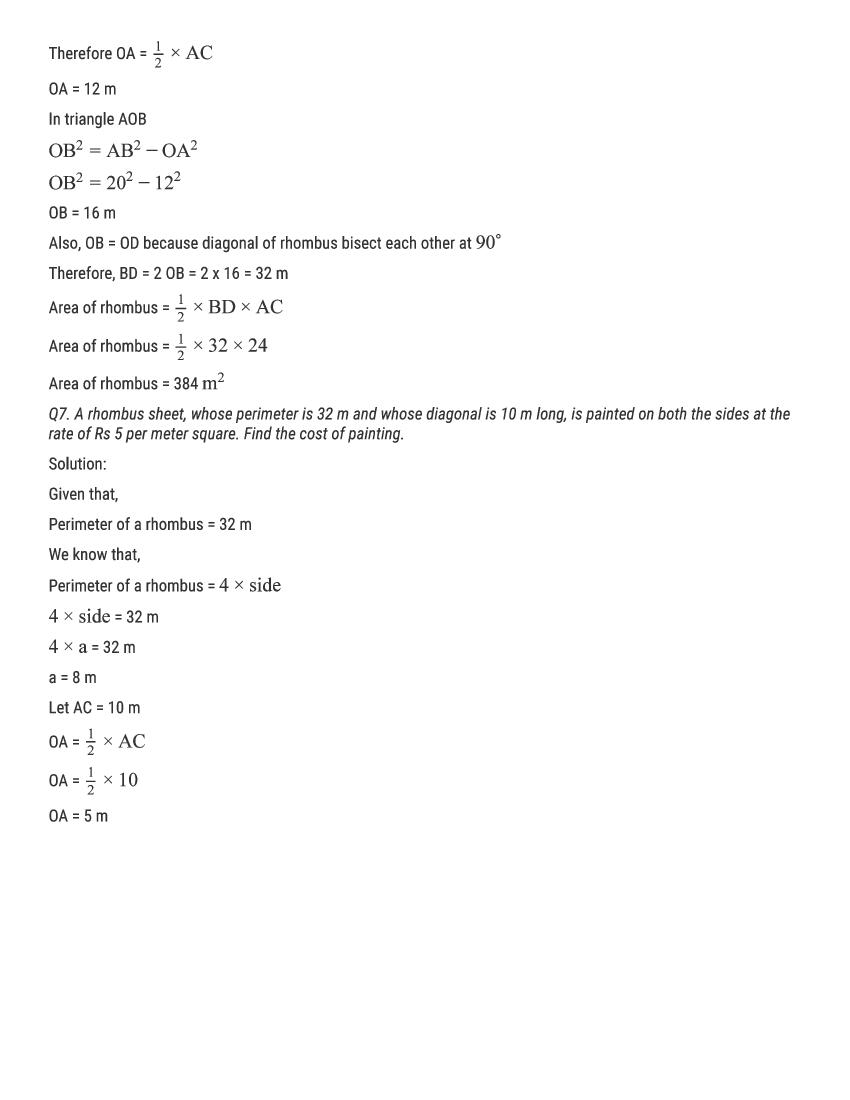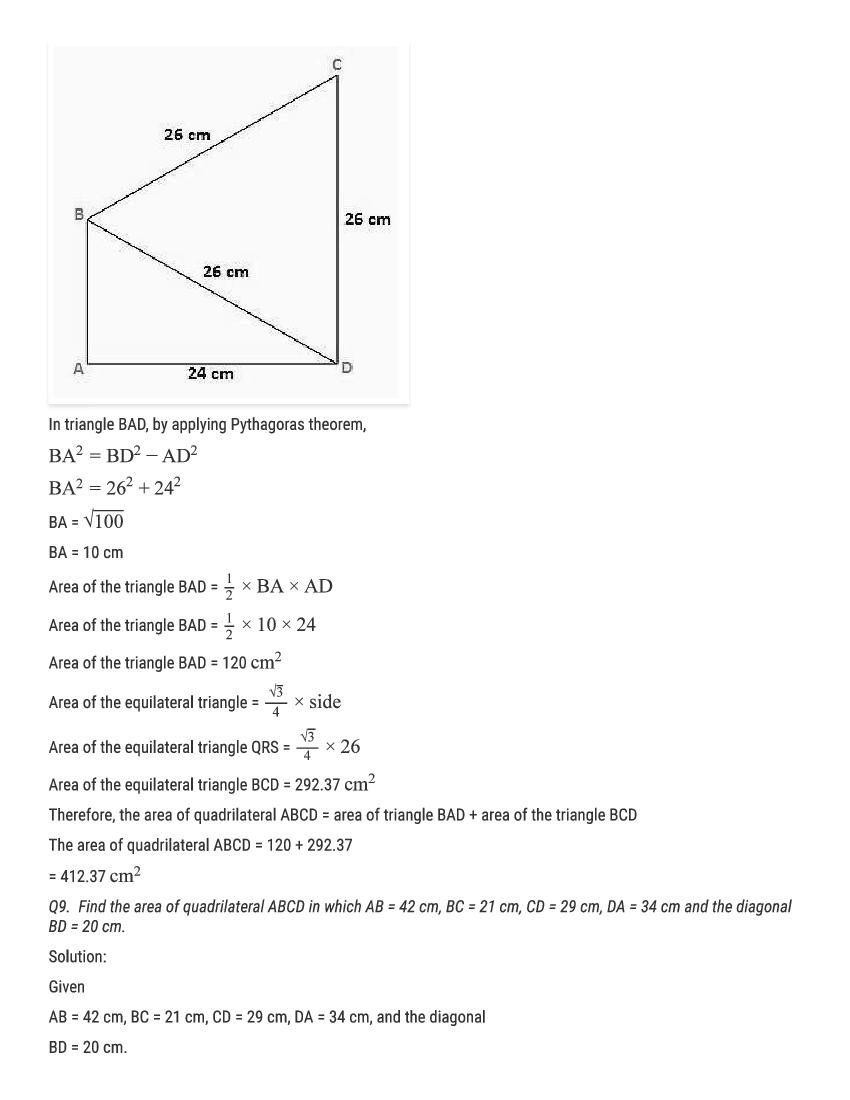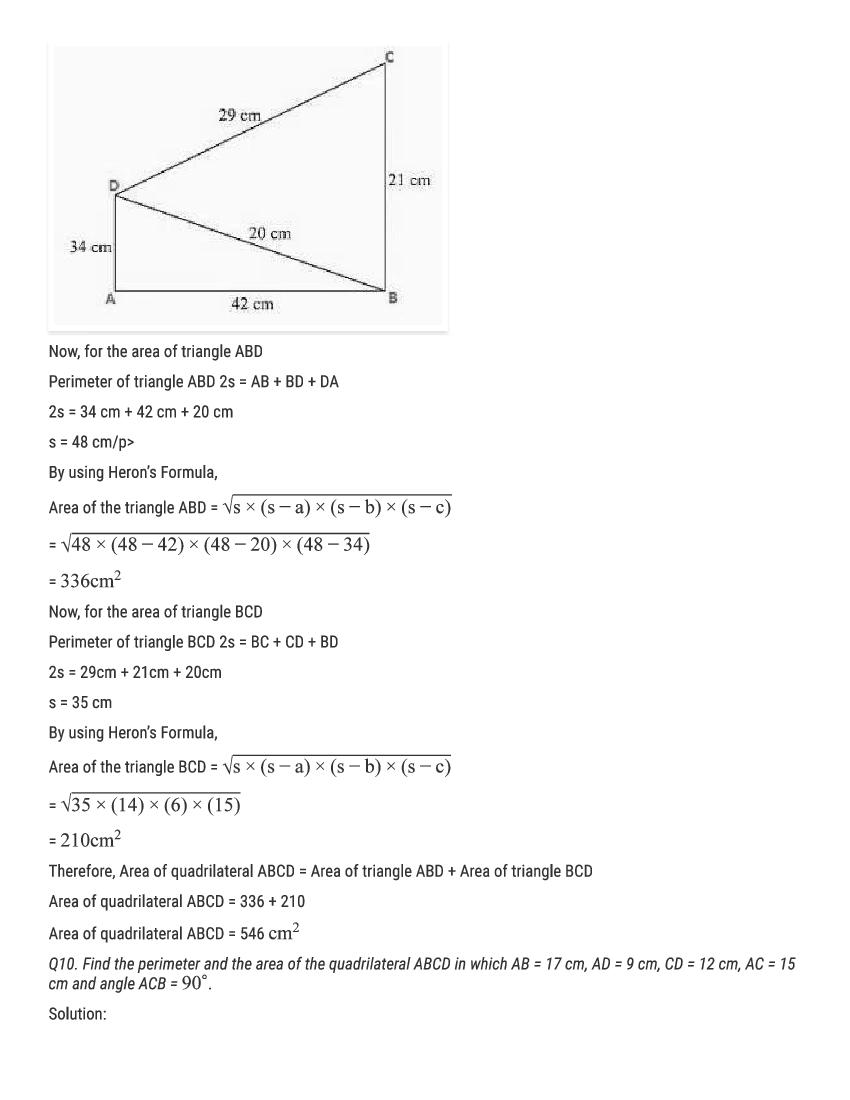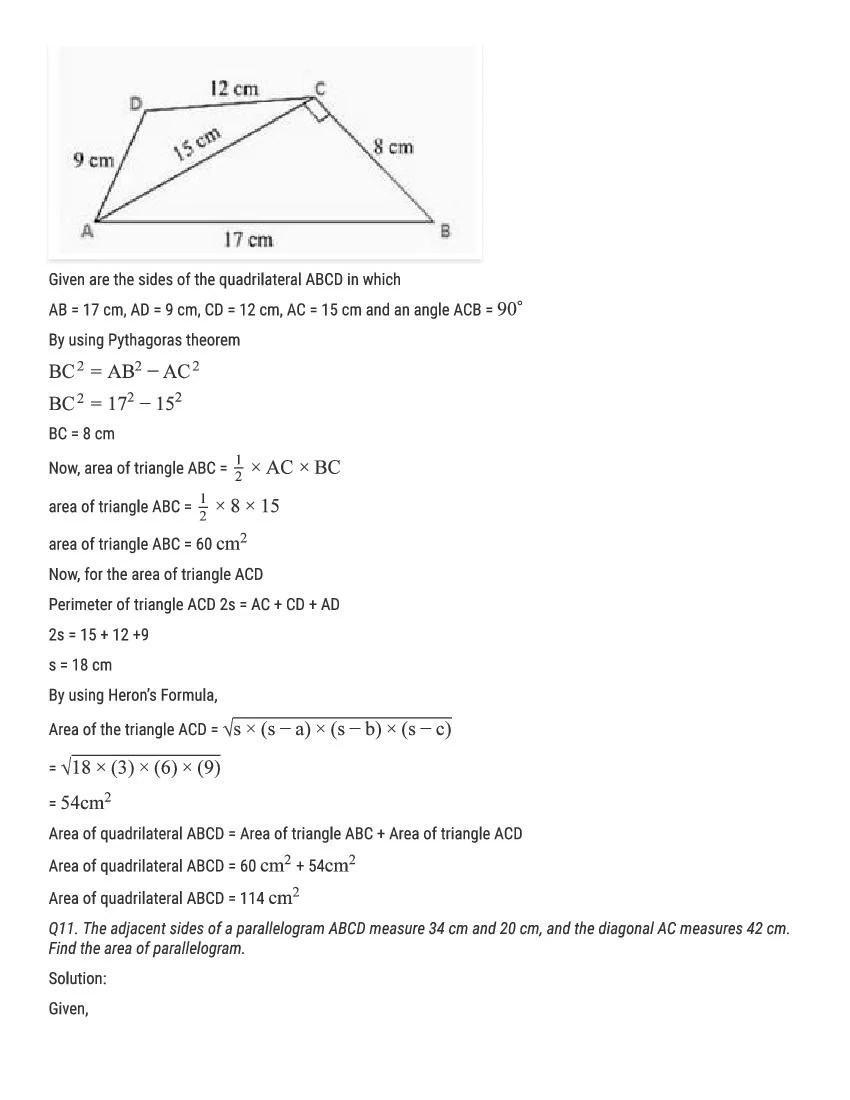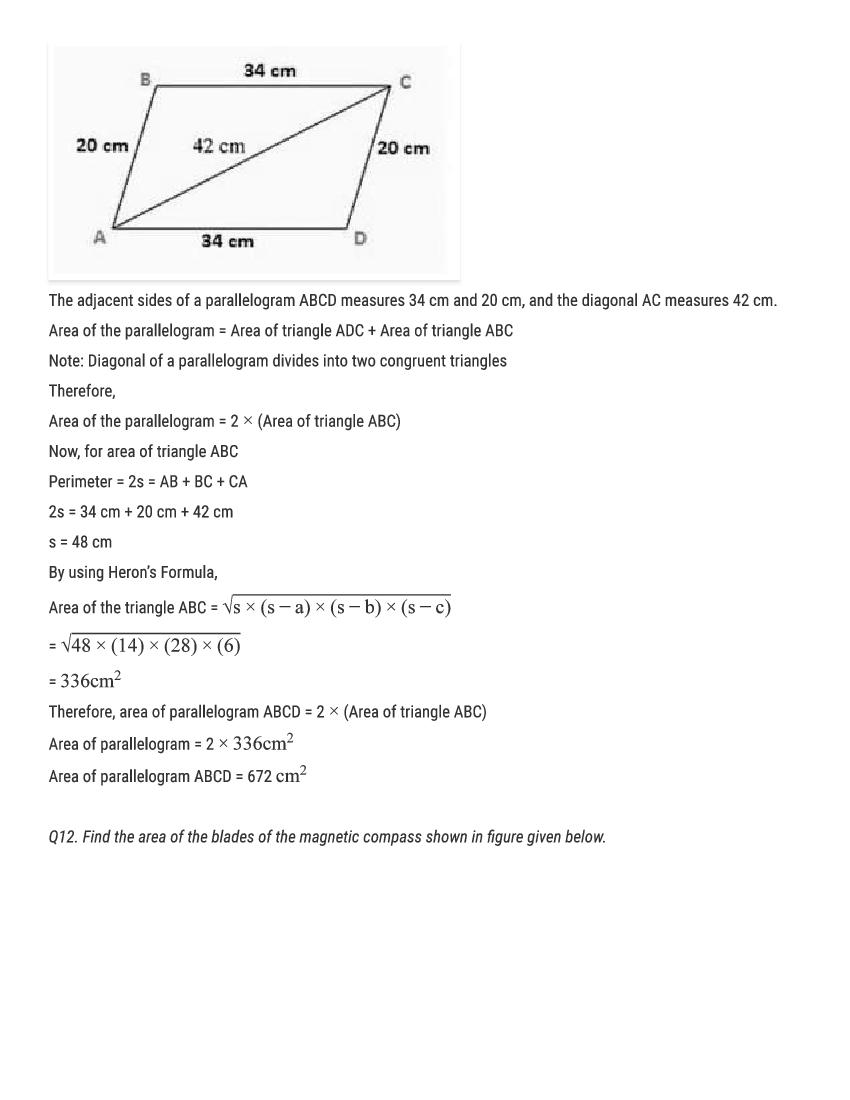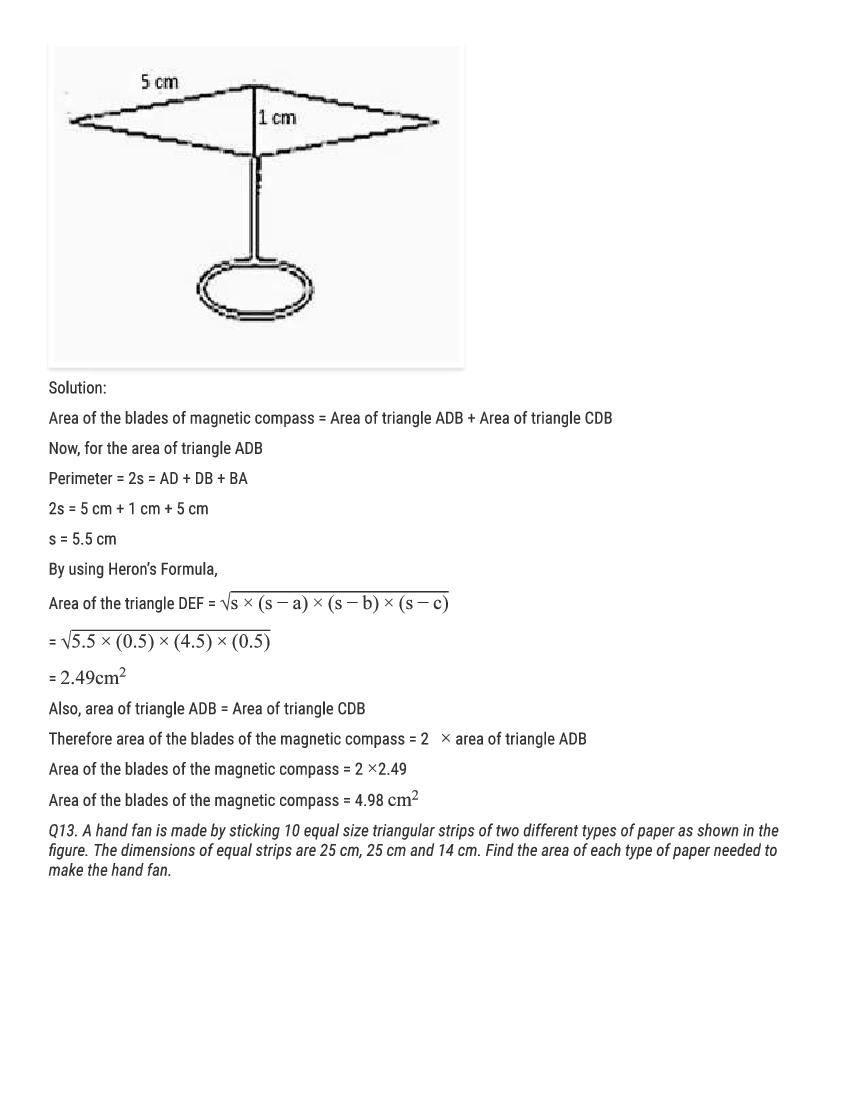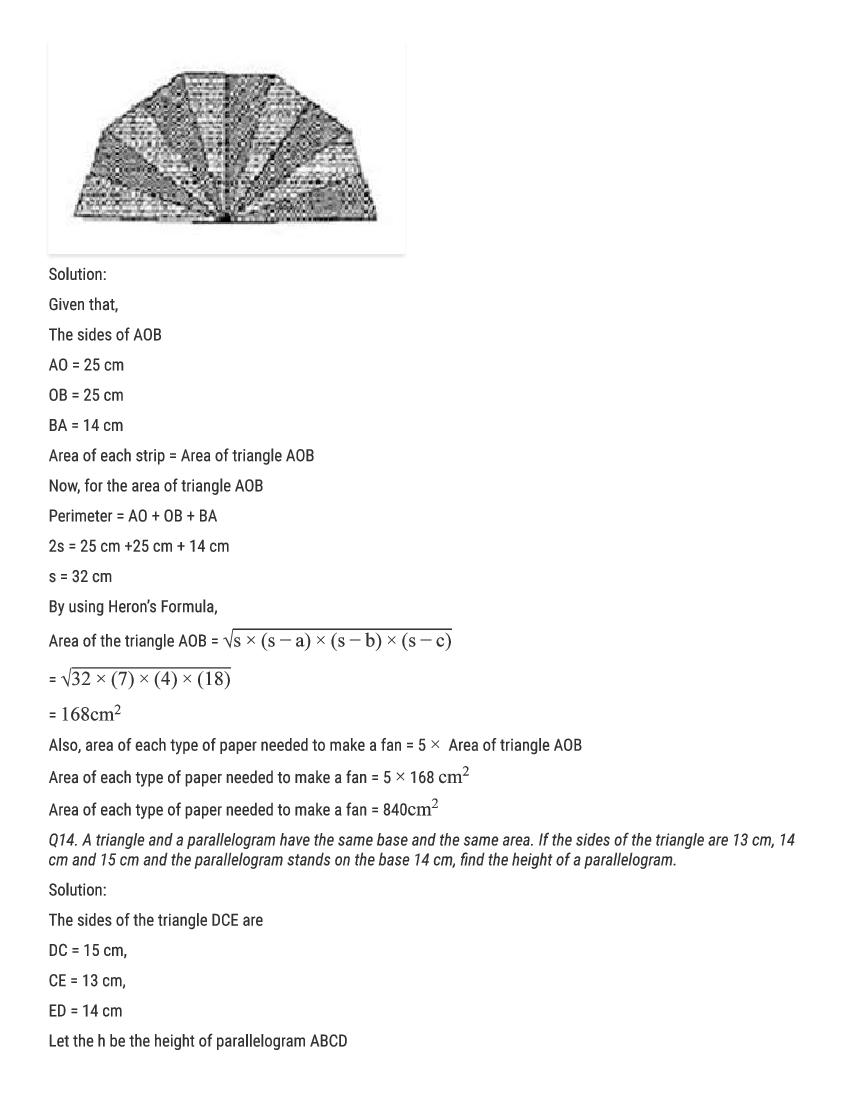# RD Sharma Solutions Class 9 Chapter 12 Heron’s Formula Exercise 12.2

Here you can get free RD Sharma Solutions for Class 9 Maths Chapter 12 Heron’s Formula Exercise 12.2. All RD Sharma Book Solutions are given here exercise wise for the chapter Heron’s Formula. RD Sharma Solutions are helpful in the preparation of several school level, graduate and undergraduate level competitive exams. Practicing questions from RD Sharma Mathematics Solutions for Class 9 Chapter 12 Heron’s Formula is proven to enhance your math skills.

 Class: Class 9th Chapter: Chapter 12 Exercise: Exercise 12.2 Name: Heron’s Formula

## RD Sharma Solutions Class 9 Chapter 12 Heron’s Formula Exercise 12.2

RD Sharma Class 9 Solutions Chapter 12 for Heron’s Formula Exercise 12.2 are given below.

RD Sharma Solutions Class 9 Chapter 12 Herons Forumula Excercise 12.2